# The Dink Network

## SlipDink's Profile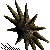SlipDink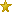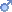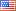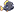2nd generation. No easy way to be free.
They once paid me to code for a living. But I'd rather 'dink around' all day.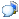Private Message

2018-10-20 17:27:13SlipDink2nd generation. No easy way to be free.
Okay, so toof.c (see this below variant of your DinkC code), now runs in DinkC; but how do we set, reset and test various bits within a DinkC integer using your code? I must be missing something here.

```// This is a variant of toof's DinkC "simple do-while loop" DinkC code:
void binary()
{
int &counter = &arg1;
debug("toof: counter=&counter; status_bd=&status_bd");
int &crap = &status_bd;
// For my tests, I used &status_bd as the global variable.
// Since it is accessible everywhere, it is not passed in.
loop:
if(&counter > 0)
{
&crap / 2;
// I assumed you meant to use the passed in value &counter
// here, not the (apparently undeclared) value &count.
&counter -= 1;
debug("toof: counter=&counter; crap=&crap");
wait(200);
goto loop;
}
else
{
// I found the DinkC modulo math function in
// the "DinkC Reference v4.0" help file! I also
// changed the code to return a value via normal
// DinkC methods instead of the slightly more
// efficient method (but *somewhat* less safe (?)
// if more than one script is calling this code at
// about the same time?) of using a global to
// return the value.
&crap = math_mod(&crap);
return(&crap);
}
}
```

For example, if we set &status_bd to 12 and pass in the value 8 as the &counter parameter, it would be nice if we got back some indication that the "bit 3 (value 8)" is set in &status_bd. We invoked "external("toof", "binary", 8);". Below are the results we find in DEBUG.TXT.

```Dink:toof: counter=8; status_bd=12
Dink:toof: counter=7; crap=6
Dink:toof: counter=6; crap=3
Dink:toof: counter=5; crap=1
Dink:toof: counter=4; crap=0
Dink:toof: counter=3; crap=0
Dink:toof: counter=2; crap=0
Dink:toof: counter=1; crap=0
Dink:toof: counter=0; crap=0
```

If we do the same thing for &status_bd (setting it to 12) and invoke "" and then check "bit 3 (value 8)", we get results like those show below which tells us that bit 8 is set, as we would expect.

```Dink:CHKBIT: do operation(-1) on bd=12(2: status_bd) using which=8)
Dink:CHKBIT: do operation(-1) using which=8; wrkbit=1073741824)
Dink:CHKBIT: op:-1 --> bs=12; which=8; wrkbit=536870912
Dink:CHKBIT: op:-1 --> bs=12; which=8; wrkbit=268435456
Dink:CHKBIT: op:-1 --> bs=12; which=8; wrkbit=134217728
Dink:CHKBIT: op:-1 --> bs=12; which=8; wrkbit=67108864
Dink:CHKBIT: op:-1 --> bs=12; which=8; wrkbit=33554432
Dink:CHKBIT: op:-1 --> bs=12; which=8; wrkbit=16777216
Dink:CHKBIT: op:-1 --> bs=12; which=8; wrkbit=8388608
Dink:CHKBIT: op:-1 --> bs=12; which=8; wrkbit=4194304
Dink:CHKBIT: op:-1 --> bs=12; which=8; wrkbit=2097152
Dink:CHKBIT: op:-1 --> bs=12; which=8; wrkbit=1048576
Dink:CHKBIT: op:-1 --> bs=12; which=8; wrkbit=524288
Dink:CHKBIT: op:-1 --> bs=12; which=8; wrkbit=262144
Dink:CHKBIT: op:-1 --> bs=12; which=8; wrkbit=131072
Dink:CHKBIT: op:-1 --> bs=12; which=8; wrkbit=65536
Dink:CHKBIT: op:-1 --> bs=12; which=8; wrkbit=32768
Dink:CHKBIT: op:-1 --> bs=12; which=8; wrkbit=16384
Dink:CHKBIT: op:-1 --> bs=12; which=8; wrkbit=8192
Dink:CHKBIT: op:-1 --> bs=12; which=8; wrkbit=4096
Dink:CHKBIT: op:-1 --> bs=12; which=8; wrkbit=2048
Dink:CHKBIT: op:-1 --> bs=12; which=8; wrkbit=1024
Dink:CHKBIT: op:-1 --> bs=12; which=8; wrkbit=512
Dink:CHKBIT: op:-1 --> bs=12; which=8; wrkbit=256
Dink:CHKBIT: op:-1 --> bs=12; which=8; wrkbit=128
Dink:CHKBIT: op:-1 --> bs=12; which=8; wrkbit=64
Dink:CHKBIT: op:-1 --> bs=12; which=8; wrkbit=32
Dink:CHKBIT: op:-1 --> bs=12; which=8; wrkbit=16
Dink:CHKBIT: op:-1 --> bs=12; which=8; wrkbit=8
Dink:CHKBIT: op:query --> bit 8 was found set in 12.
Dink:CHKBIT: done
```

### SlipDink has released 8 files

TitleCategoryAvgUpdated
A Little AdventureD-Mod, RompN/AAugust 2nd, 2023
Periculo IslandD-Mod, QuestN/AMay 20th, 2023
Checkbit Development, SourceN/AJune 21st, 2022
All that Glitters!D-Mod, Quest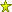7.2February 26th, 2021
Before...D-Mod, QuestN/AApril 13th, 2020
Crowns of StoneD-Mod, Quest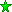9.1March 8th, 2020
THE LAST QUEST (part 2): The Iron Ring of DestinyD-Mod, Quest8.7December 7th, 2019
The Last Quest Part 1: The OraculumD-Mod, Romp8.2December 4th, 2019

### SlipDink has written 5 reviews

TitleFileTypeScoreDate
The best that can fairly be said about this dmod. An Ancient Voice: A Tale of Four SorrowsNormal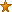3.2September 19th, 2020
The Golden Duck is a bar I'd like to visit more often! Golden DuckNormal8.7December 5th, 2018
Great game in the spirit of Bloop! Bloop's PurpoiseNormal9.9June 19th, 2017
The 2nd best entry in the June 2017 Bloop the Fish contest! Another Fish Named BloopNormal9.7June 19th, 2017
An entertaining, though somewhat flawed effort The Black SkullFeatured7.9November 14th, 2016